PROC STEPDISC Statement

PROC STEPDISC <options> ;

The PROC STEPDISC statement invokes the STEPDISC procedure. The options listed in Table 85.1 are available in the PROC STEPDISC statement.

Table 85.1 STEPDISC Procedure Options

Option

Description

Input Data Set

Specifies input SAS data set

Method Details

Specifies maximum macro variable lists

Specifies method

Specifies singularity

Control Stepwise Selection

Specifies entry significance

Specifies staying significance

Specifies entry partial R square

Specifies staying partial R square

Forces inclusion of variables

Specifies maximum number of steps

Specifies variables to begin

Specifies number of variables in final model

Control Displayed Output

Displays all

Displays between correlations

Displays between covariances

Displays between SSCPs

Displays pooled correlations

Displays pooled covariances

Displays pooled SSCPs

Suppresses output

Displays descriptive statistics

Displays standardized class means

Displays total correlations

Displays total covariances

Displays total SSCPs

Displays within correlations

Displays within covariances

Displays within SSCPs

ALL

activates all of the display options.

BCORR

displays between-class correlations.

BCOV

displays between-class covariances. The between-class covariance matrix equals the between-class SSCP matrix divided by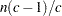, where n is the number of observations and c is the number of classes. The between-class covariances should be interpreted in comparison with the total-sample and within-class covariances, not as formal estimates of population parameters.

BSSCP

displays the between-class SSCP matrix.

DATA=SAS-data-set

specifies the data set to be analyzed. The data set can be an ordinary SAS data set or one of several specially structured data sets created by statistical procedures available with SAS/STAT software. These specially structured data sets include TYPE=CORR, COV, CSSCP, and SSCP. If the DATA= option is omitted, the procedure uses the most recently created SAS data set.

INCLUDE=n

includes the first n variables in the VAR statement in every model. By default, INCLUDE=0.

MAXMACRO=n

specifies the maximum number of macro variables with independent variable lists to create. By default, MAXMACRO=100. PROC STEPDISC saves the list of selected variables in a macro variable, &_StdVar. Suppose your input variable list consists of x1-x10; then &_StdVar would be set to x1 x3 x4 x10 if, for example, the first, third, fourth, and tenth variables were selected for the model. This list can be used, for example, in a subsequent procedure’s VAR statement as follows:

```var &_stdvar;
```

With BY processing, one macro variable is created for each BY group, and the macro variables are indexed by the BY-group number. The MAXMACRO= option can be used to either limit or increase the number of these macro variables in processing data sets with many BY groups. The macro variables are created as follows:

 With no BY processing, PROC STEPDISC creates the following: _StdVar selected variables _StdVar1 selected variables _StdNumBys number of BY groups (1) _StdNumMacroBys number of _StdVari macro variables actually made (1) With BY processing, PROC STEPDISC creates the following: _StdVar selected variables for BY group 1 _StdVar1 selected variables for BY group 1 _StdVar2 selected variables for BY group 2 . . . _StdVarm selected variables for BY group m, where a number is substituted for m _StdNumBys n, the number of BY groups _StdNumMacroBys the number m of _StdVari macro variables actually made. This value might be less than _StdNumbys = n, and it is less than or equal to the MAXMACRO= value.
MAXSTEP=n

specifies the maximum number of steps. By default, MAXSTEP= two times the number of variables in the VAR statement.

METHOD=BACKWARD | BW
METHOD=FORWARD | FW
METHOD=STEPWISE | SW

specifies the method used to select the variables in the model. The BACKWARD method specifies backward elimination, FORWARD specifies forward selection, and STEPWISE specifies stepwise selection. By default, METHOD=STEPWISE.

PCORR

displays pooled within-class correlations (partial correlations based on the pooled within-class covariances).

PCOV

displays pooled within-class covariances.

PR2ENTRY=p
PR2E=p

specifies the partial R square for adding variables in the forward selection mode, where
.

PR2STAY=p
PR2S=p

specifies the partial R square for retaining variables in the backward elimination mode, where.

PSSCP

displays the pooled within-class corrected SSCP matrix.

SHORT

suppresses the displayed output from each step.

SIMPLE

displays simple descriptive statistics for the total sample and within each class.

SINGULAR=p

specifies the singularity criterion for entering variables, where
. PROC STEPDISC precludes the entry of a variable if the squared multiple correlation of the variable with the variables already in the model exceeds
. With more than one variable already in the model, PROC STEPDISC also excludes a variable if it would cause any of the variables already in the model to have a squared multiple correlation (with the entering variable and the other variables in the model) exceeding. By default, SINGULAR= 1E–8.

SLENTRY=p
SLE=p

specifies the significance level for adding variables in the forward selection mode, where
. The default value is 0.15.

SLSTAY=p
SLS=p

specifies the significance level for retaining variables in the backward elimination mode, where. The default value is 0.15.

START=n

specifies that the first n variables in the VAR statement be used to begin the selection process. When you specify METHOD=FORWARD or METHOD=STEPWISE, the default value is 0; when you specify METHOD=BACKWARD, the default value is the number of variables in the VAR statement.

STDMEAN

displays total-sample and pooled within-class standardized class means.

STOP=n

specifies the number of variables in the final model. The STEPDISC procedure stops the selection process when a model with n variables is found. This option applies only when you specify METHOD=FORWARD or METHOD=BACKWARD. When you specify METHOD=FORWARD, the default value is the number of variables in the VAR statement; when you specify METHOD=BACKWARD, the default value is 0.

TCORR

displays total-sample correlations.

TCOV

displays total-sample covariances.

TSSCP

displays the total-sample corrected SSCP matrix.

WCORR

displays within-class correlations for each class level.

WCOV

displays within-class covariances for each class level.

WSSCP

displays the within-class corrected SSCP matrix for each class level.